# PHIL 201 CHAPTER 7 PROPOSITIONAL LOGIC Propositional Logic

• Slides: 42PHIL 201 CHAPTER 7 PROPOSITIONAL LOGICPropositional Logic Though working with categorical logic seems to involve us in language and argument forms that aren’t very common in our everyday lives, this appearance is misleading. ◦ Not only can most ordinary language statements be given a categorical interpretation (rewriting them as categorical propositions), but many arguments that are not apparently syllogisms can be appropriately interpreted as chains of syllogisms. However, there a number of arguments for which categorical analysis seems to give counter -intuitive results (arguments which seem clearly valid, but which from the categorical standpoint are judged invalid). Recognizing this and other limitations, logicians developed a new logical framework: propositional logic. Some of the obvious differences between propositional logic and categorical logic include: ◦ ◦ PL provides precise definitions for sentences containing and, or, not, if, and only if. PL captures much more of ordinary language than is possible with CL. PL’s basic elements are statements rather than classes. Unlike A, E, I, O, contains an unlimited number of complex statements.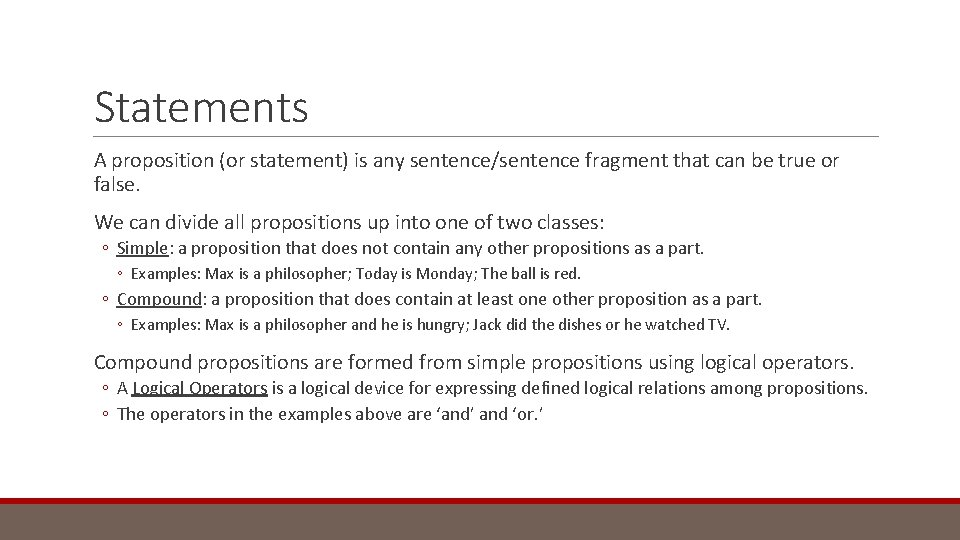Statements A proposition (or statement) is any sentence/sentence fragment that can be true or false. We can divide all propositions up into one of two classes: ◦ Simple: a proposition that does not contain any other propositions as a part. ◦ Examples: Max is a philosopher; Today is Monday; The ball is red. ◦ Compound: a proposition that does contain at least one other proposition as a part. ◦ Examples: Max is a philosopher and he is hungry; Jack did the dishes or he watched TV. Compound propositions are formed from simple propositions using logical operators. ◦ A Logical Operators is a logical device for expressing defined logical relations among propositions. ◦ The operators in the examples above are ‘and’ and ‘or. ’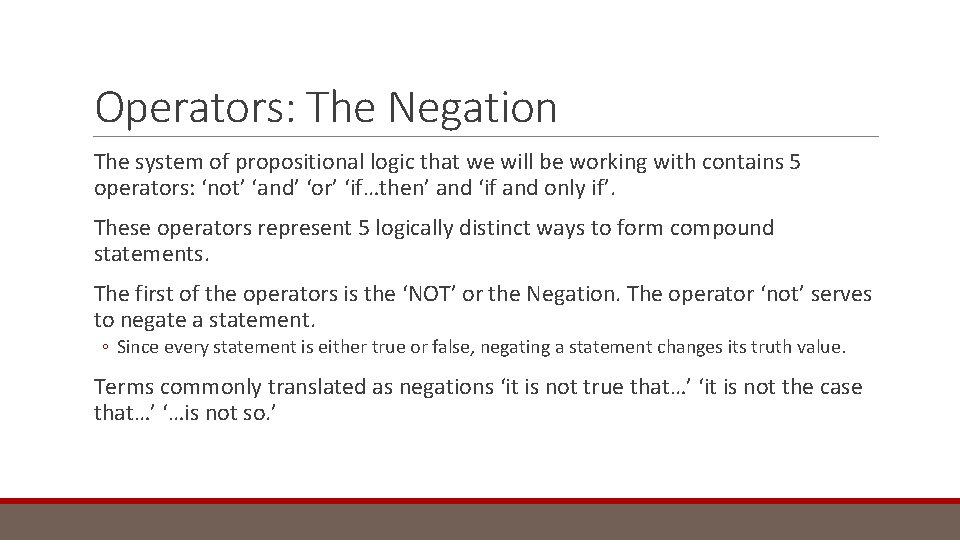Operators: The Negation The system of propositional logic that we will be working with contains 5 operators: ‘not’ ‘and’ ‘or’ ‘if…then’ and ‘if and only if’. These operators represent 5 logically distinct ways to form compound statements. The first of the operators is the ‘NOT’ or the Negation. The operator ‘not’ serves to negate a statement. ◦ Since every statement is either true or false, negating a statement changes its truth value. Terms commonly translated as negations ‘it is not true that…’ ‘it is not the case that…’ ‘…is not so. ’Operators: The Conjunction and The Disjunction The operator ‘and’ is used to form compound statements called conjunctions. ◦ A conjunction asserts that the two statements conjoined (the conjuncts) are both true. Terms commonly translated as conjunctions: ‘and’ ‘but’ ‘yet’ ‘nevertheless. ’ The operator ‘or’ is used to form compounds called disjunctions. ◦ A disjunction says that at least one of the two statements of the compound (the disjuncts) is true. ◦ The disjunction we will be using is called the ‘inclusive’ disjunction because it allows for both of the disjuncts to be true. ◦ Another interpretation of the disjunction is called the ‘exclusive’ because it insists that one and only one of the disjuncts is true. ◦ In English we use both senses (though the inclusive one is more common) so later on we will learn a way to translate the exclusive disjunction using the operators we have available to us. ◦ Unless it is specified, assume that a disjunction is an inclusive one ‘p is true or q is true or both are true’.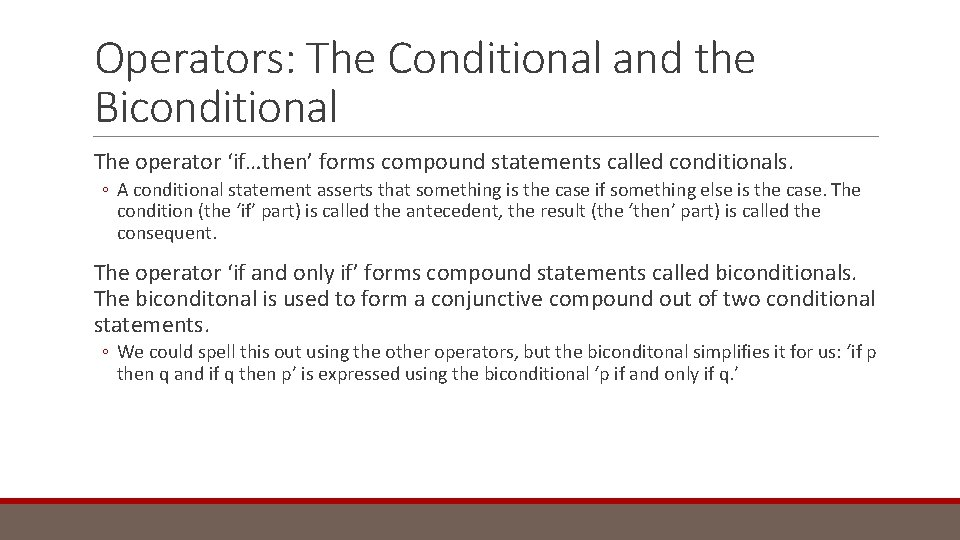Operators: The Conditional and the Biconditional The operator ‘if…then’ forms compound statements called conditionals. ◦ A conditional statement asserts that something is the case if something else is the case. The condition (the ‘if’ part) is called the antecedent, the result (the ‘then’ part) is called the consequent. The operator ‘if and only if’ forms compound statements called biconditionals. The biconditonal is used to form a conjunctive compound out of two conditional statements. ◦ We could spell this out using the other operators, but the biconditonal simplifies it for us: ‘if p then q and if q then p’ is expressed using the biconditional ‘p if and only if q. ’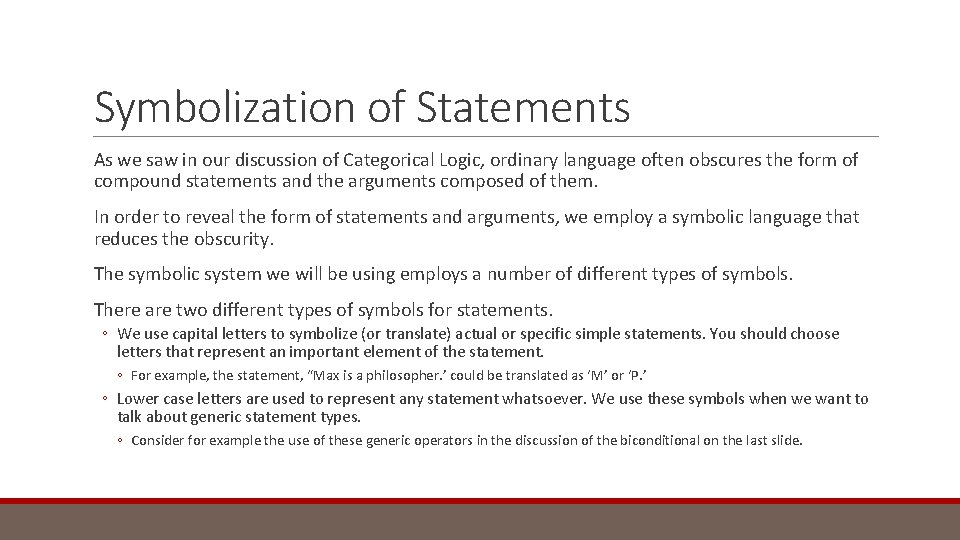Symbolization of Statements As we saw in our discussion of Categorical Logic, ordinary language often obscures the form of compound statements and the arguments composed of them. In order to reveal the form of statements and arguments, we employ a symbolic language that reduces the obscurity. The symbolic system we will be using employs a number of different types of symbols. There are two different types of symbols for statements. ◦ We use capital letters to symbolize (or translate) actual or specific simple statements. You should choose letters that represent an important element of the statement. ◦ For example, the statement, “Max is a philosopher. ’ could be translated as ‘M’ or ‘P. ’ ◦ Lower case letters are used to represent any statement whatsoever. We use these symbols when we want to talk about generic statement types. ◦ Consider for example the use of these generic operators in the discussion of the biconditional on the last slide.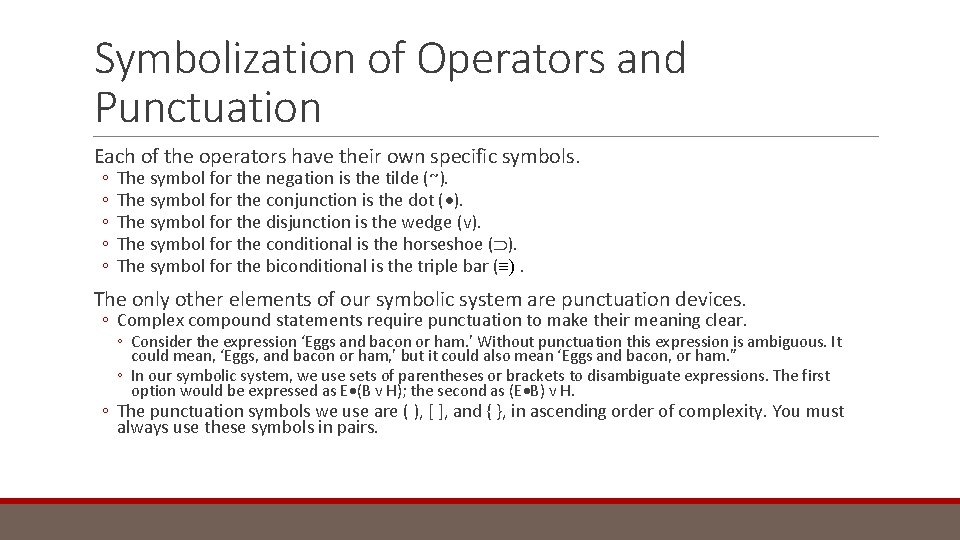Symbolization of Operators and Punctuation Each of the operators have their own specific symbols. ◦ ◦ ◦ The symbol for the negation is the tilde (~). The symbol for the conjunction is the dot ( ). The symbol for the disjunction is the wedge (v). The symbol for the conditional is the horseshoe ( ). The symbol for the biconditional is the triple bar (º). The only other elements of our symbolic system are punctuation devices. ◦ Complex compound statements require punctuation to make their meaning clear. ◦ Consider the expression ‘Eggs and bacon or ham. ’ Without punctuation this expression is ambiguous. It could mean, ‘Eggs, and bacon or ham, ’ but it could also mean ‘Eggs and bacon, or ham. ” ◦ In our symbolic system, we use sets of parentheses or brackets to disambiguate expressions. The first option would be expressed as E (B v H); the second as (E B) v H. ◦ The punctuation symbols we use are ( ), [ ], and { }, in ascending order of complexity. You must always use these symbols in pairs.Symbolization of Statements We can translate any natural language statement, simple or compound, into our symbolic system using these symbols. The purpose of symbolization is to reveal the form of the statement and prepare it for logical analysis. There are four steps for any symbolization: 1. 2. 3. 4. Identify the simple statement or statements. Choose capital letters to symbolize the statements. Choose letters that are reflective of the statement being symbolized and apply your symbolization consistently. For compound statements, supply the appropriate symbol for the logical operator Use the punctuation symbols to make your translation unambiguous.Translating Tips: Negations, Conjunctions and Disjunctions The scope of a negation is whatever immediately follows it. ◦ If what follows is a simple, the negation operates only on that simple. If what follows is a punctuated compound (A v B) the negation operates on the entire compound. Variations on the Conjunction and Disjunction 1. ‘neither p nor q’; ‘both p and q are not’ etc. —translate as either ~(p v q) or ~p ~q. 2. ‘not both p and q’; ‘not p or not q’ etc. —translate as either ~(p q) or ~p v ~q. 3. ‘either p or q, but not both’ (the exclusive disjunction—see above)—translate as (p v q) + ~(p + q).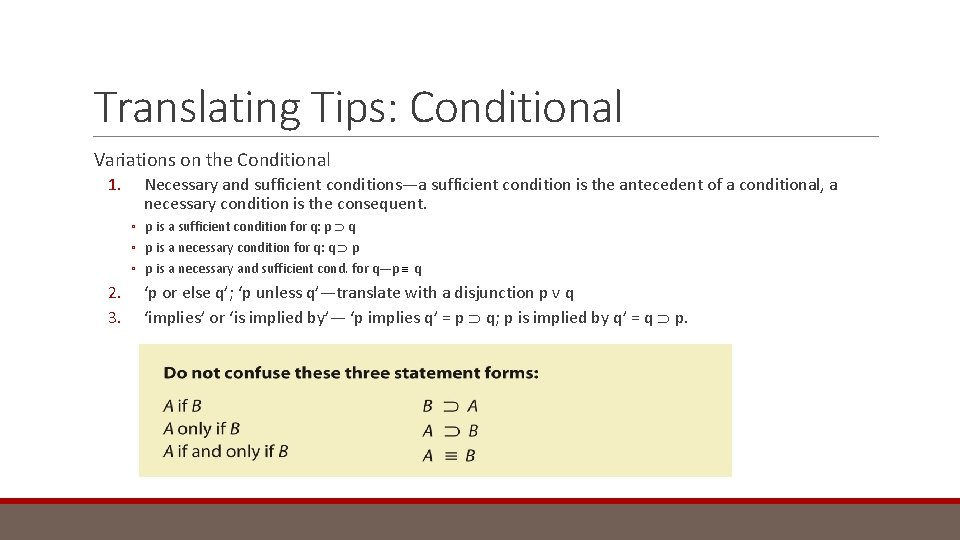Translating Tips: Conditional Variations on the Conditional 1. Necessary and sufficient conditions—a sufficient condition is the antecedent of a conditional, a necessary condition is the consequent. ◦ p is a sufficient condition for q: p q ◦ p is a necessary condition for q: q p ◦ p is a necessary and sufficient cond. for q—p º q 2. 3. ‘p or else q’; ‘p unless q’—translate with a disjunction p v q ‘implies’ or ‘is implied by’— ‘p implies q’ = p q; p is implied by q’ = q p.Exercise 7 A Example ◦ I will stop by to visit only if I have finished my homework. Answer: ◦ Let V = I will stop by to visit, and H = I have finished my homework. ◦ V only if H ◦ V H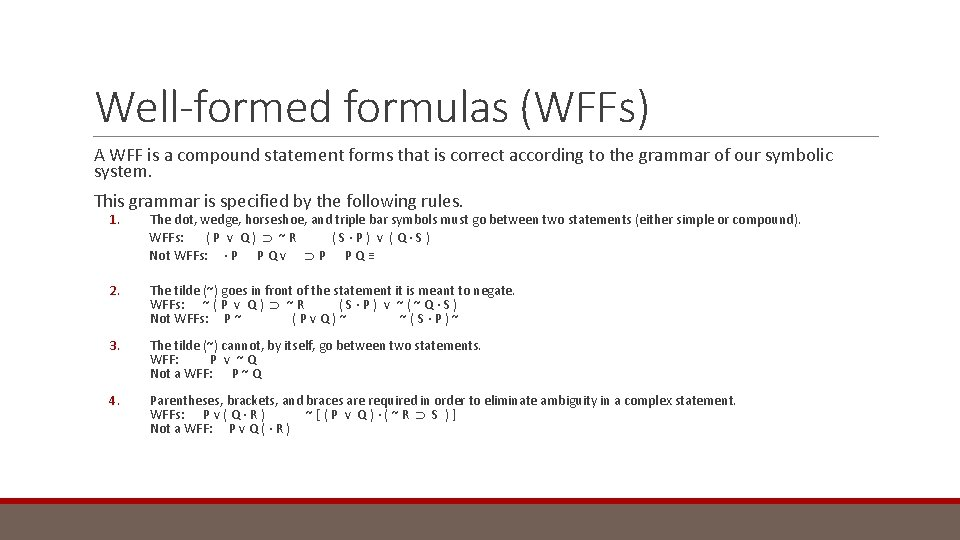Well-formed formulas (WFFs) A WFF is a compound statement forms that is correct according to the grammar of our symbolic system. This grammar is specified by the following rules. 1. The dot, wedge, horseshoe, and triple bar symbols must go between two statements (either simple or compound). WFFs: (P v Q) ~R (S·P) v (Q·S) Not WFFs: · P P Q v P P Q ≡ 2. The tilde (~) goes in front of the statement it is meant to negate. WFFs: ~ ( P v Q ) ~ R (S·P) v ~(~Q·S) Not WFFs: P ~ (Pv. Q)~ ~(S·P)~ 3. The tilde (~) cannot, by itself, go between two statements. WFF: P v ~Q Not a WFF: P ~ Q 4. Parentheses, brackets, and braces are required in order to eliminate ambiguity in a complex statement. WFFs: P v ( Q · R ) ~[(P v Q)·(~R S )] Not a WFF: P v Q ( · R )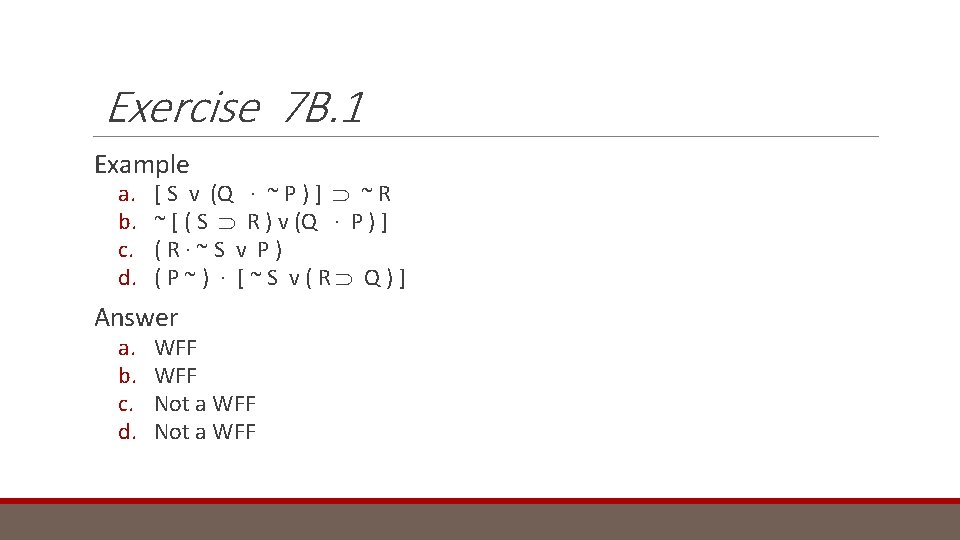Exercise 7 B. 1 Example a. b. c. d. [ S v (Q · ~ P ) ] ~ R ~ [ ( S R ) v (Q · P ) ] (R·~S v P) (P~) · [~S v(R Q)] Answer a. b. c. d. WFF Not a WFFMain Operators When translating complex compound statements (compounds with more than one operator) it is important to identify the main operator. ◦ The main operator is the operator which has as its scope everything else in the statement. It specifies what kind of compound the statement is.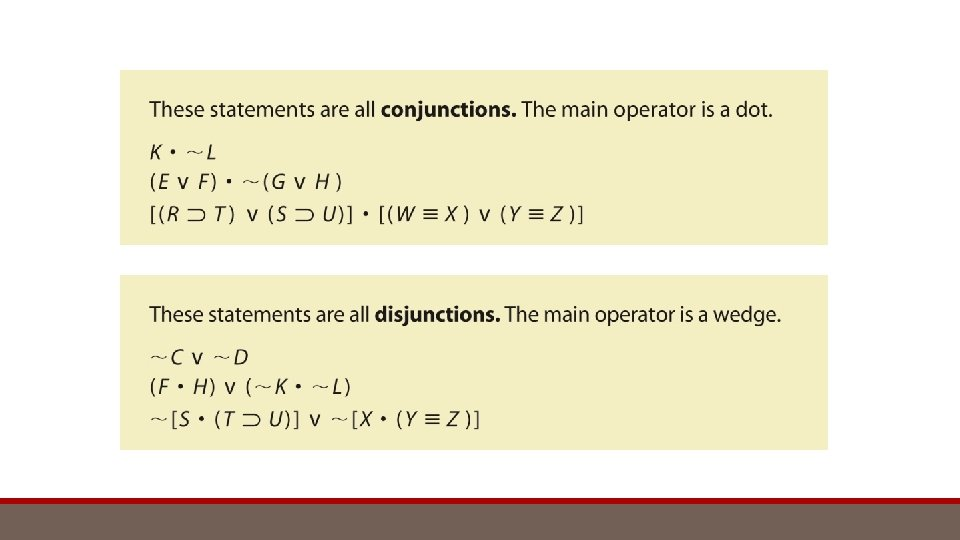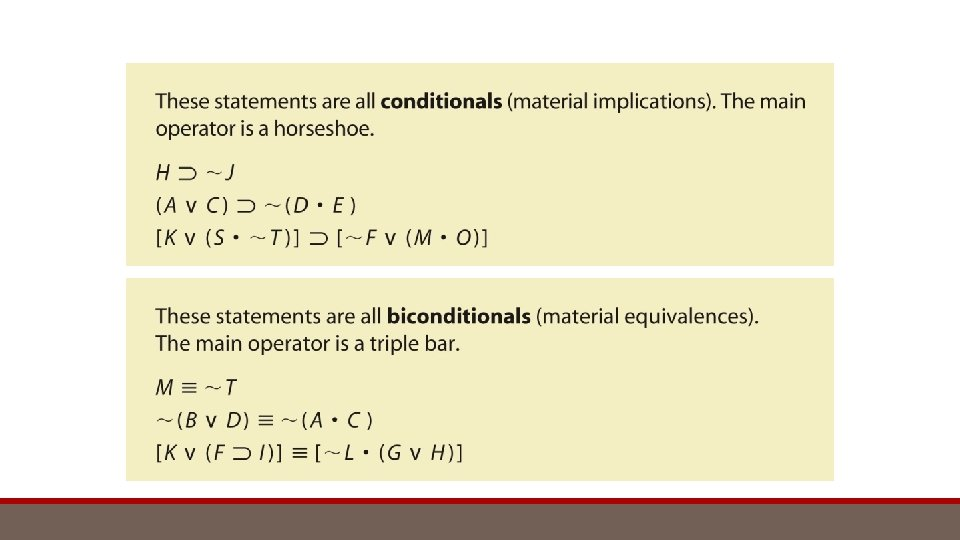Exercises 7 B. 2 Example ◦ ~[(A ·C) (B v D)] Answer ◦ The main operator is the (~) sign. Example ◦ [(A · C) ~B ] v D Answer ◦ The main operator is the (v) sign.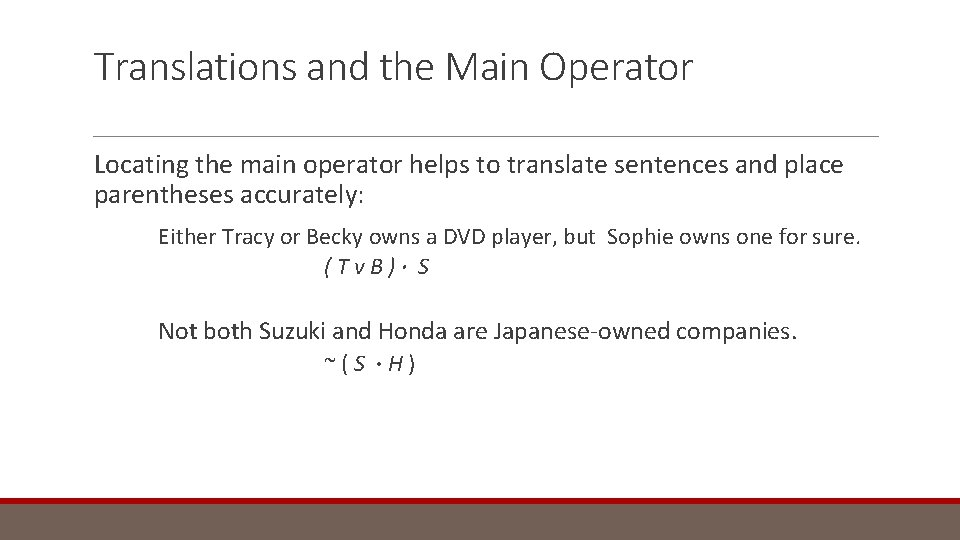Translations and the Main Operator Locating the main operator helps to translate sentences and place parentheses accurately: Either Tracy or Becky owns a DVD player, but Sophie owns one for sure. (Tv. B)· S Not both Suzuki and Honda are Japanese-owned companies. ~(S ·H)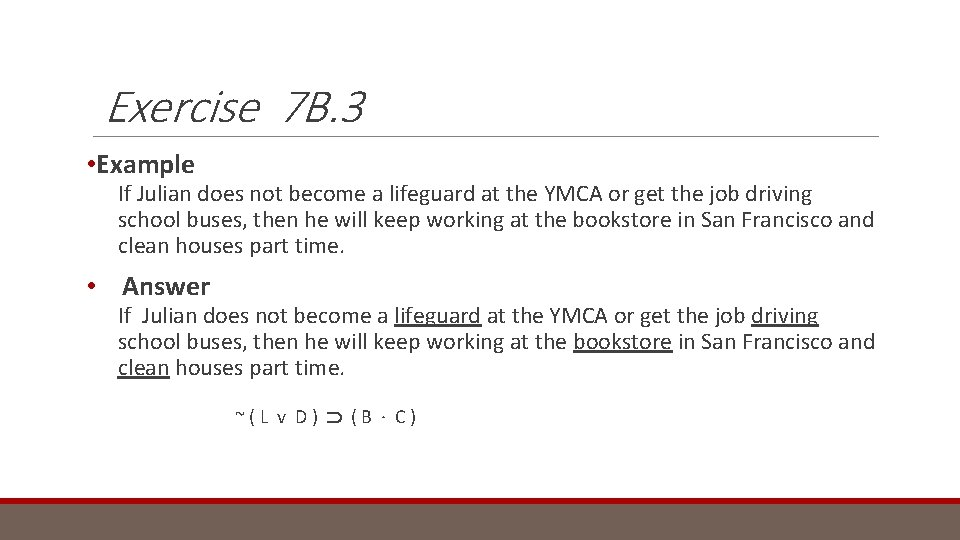Exercise 7 B. 3 • Example If Julian does not become a lifeguard at the YMCA or get the job driving school buses, then he will keep working at the bookstore in San Francisco and clean houses part time. • Answer If Julian does not become a lifeguard at the YMCA or get the job driving school buses, then he will keep working at the bookstore in San Francisco and clean houses part time. ~(L v D) (B · C)Truth-Functional Compounds Compound statements formed by our operators are called a truth-functional compounds because the truth value of the compound is determined by the truth value of the simple statements that make up the compound in conjunction with the operators. ◦ Truth Functionality: When a truth value of a compound proposition is determined by the truth values of its components and by the logical operators. The notion of truth functionality allows us to specify the truth-functional definitions of our operators. ◦ Truth Functional Definition: How an operator specifies the truth value of a compound given specified truth values of the simples that make up the compound. We do this using a device called a truth table: a table highlighting the conditions under which a statement is true or false.Truth Functional Definitions of the Negation, Conjunction and Disjunction Negation: the truth-value of the negation is the opposite of the truth-value of the statement. We can see this in the truth table for the negation. p ~p -----T F F T Conjunction: A conjunction is true only if both of the conjuncts are true; otherwise the value of the conjunction is false. p q ---------T T F F F 3)Disjunction: A disjunction is true if at least one of the disjuncts is true; it is false if both are false. P v q -------T T T F F FTruth-Functional Definitions of the Conditional and Biconditional Conditional: A conditional is true in all cases except those in which p is true and q is false (the situation excluded by the if…then). p q -------T T F F F T T F Biconditional: A biconditional is true only when the truth-values of both conditions are the same; it’s false when they are different. p º q -------T T F F T F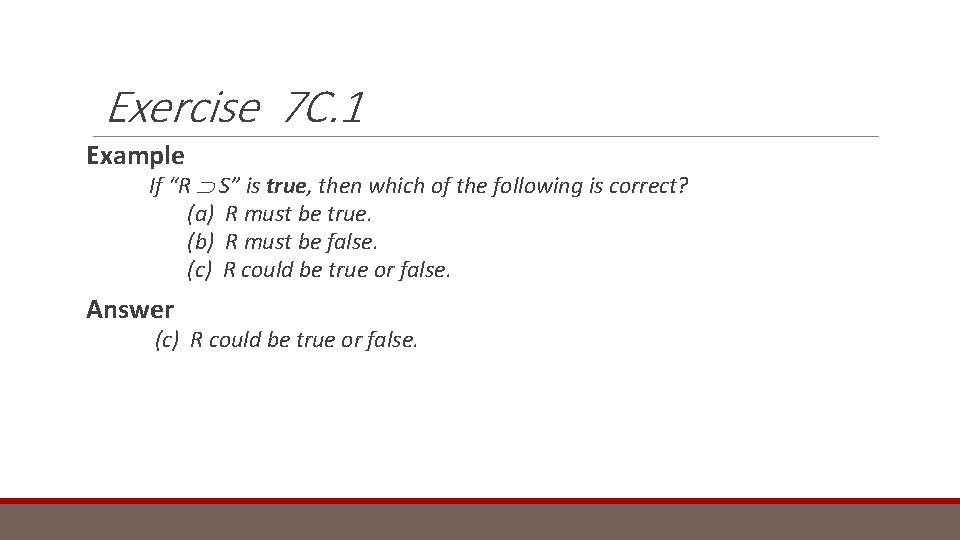Exercise 7 C. 1 Example If “R S” is true, then which of the following is correct? (a) R must be true. (b) R must be false. (c) R could be true or false. Answer (c) R could be true or false.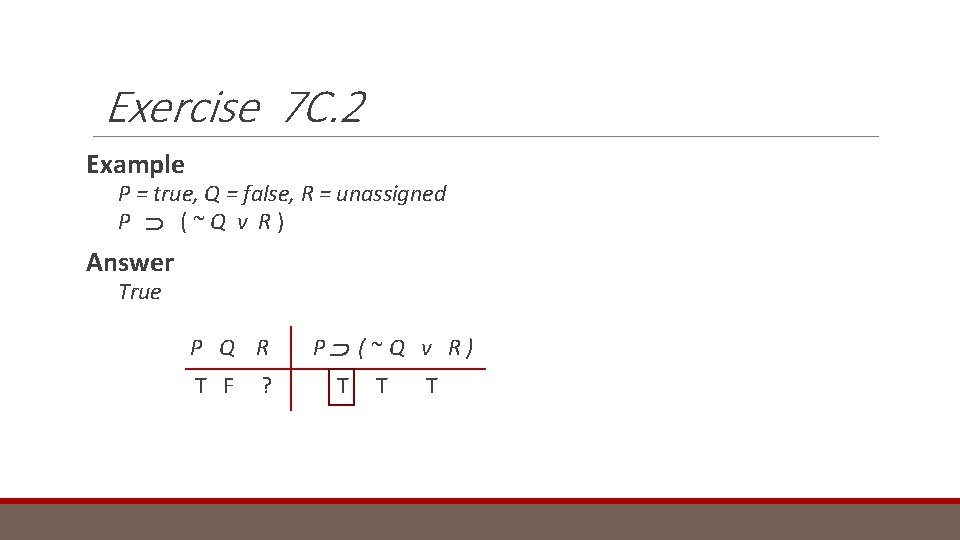Exercise 7 C. 2 Example P = true, Q = false, R = unassigned P (~Q v R) Answer True P Q R T F ? P (~Q v R) T T T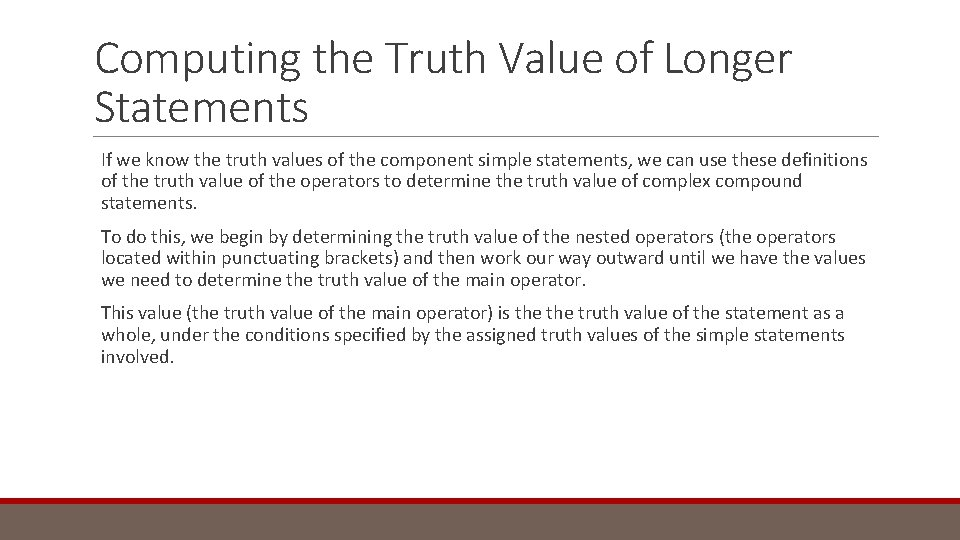Computing the Truth Value of Longer Statements If we know the truth values of the component simple statements, we can use these definitions of the truth value of the operators to determine the truth value of complex compound statements. To do this, we begin by determining the truth value of the nested operators (the operators located within punctuating brackets) and then work our way outward until we have the values we need to determine the truth value of the main operator. This value (the truth value of the main operator) is the truth value of the statement as a whole, under the conditions specified by the assigned truth values of the simple statements involved.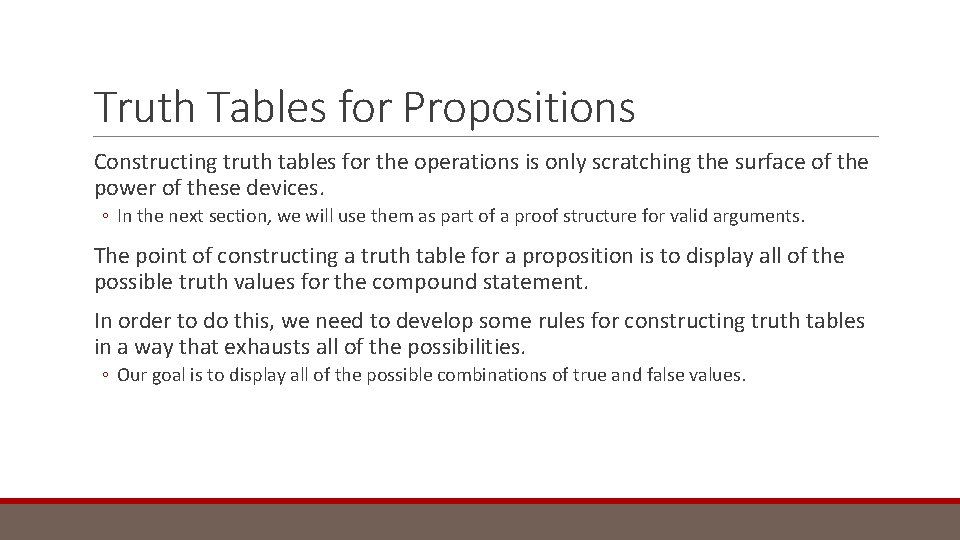Truth Tables for Propositions Constructing truth tables for the operations is only scratching the surface of the power of these devices. ◦ In the next section, we will use them as part of a proof structure for valid arguments. The point of constructing a truth table for a proposition is to display all of the possible truth values for the compound statement. In order to do this, we need to develop some rules for constructing truth tables in a way that exhausts all of the possibilities. ◦ Our goal is to display all of the possible combinations of true and false values.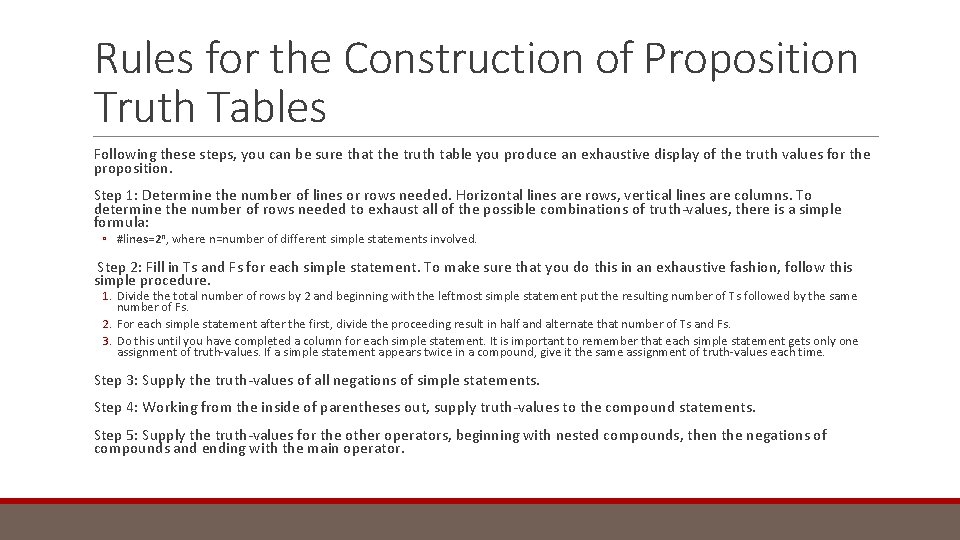Rules for the Construction of Proposition Truth Tables Following these steps, you can be sure that the truth table you produce an exhaustive display of the truth values for the proposition. Step 1: Determine the number of lines or rows needed. Horizontal lines are rows, vertical lines are columns. To determine the number of rows needed to exhaust all of the possible combinations of truth-values, there is a simple formula: ◦ #lines=2 n, where n=number of different simple statements involved. Step 2: Fill in Ts and Fs for each simple statement. To make sure that you do this in an exhaustive fashion, follow this simple procedure. 1. Divide the total number of rows by 2 and beginning with the leftmost simple statement put the resulting number of Ts followed by the same number of Fs. 2. For each simple statement after the first, divide the proceeding result in half and alternate that number of Ts and Fs. 3. Do this until you have completed a column for each simple statement. It is important to remember that each simple statement gets only one assignment of truth-values. If a simple statement appears twice in a compound, give it the same assignment of truth-values each time. Step 3: Supply the truth-values of all negations of simple statements. Step 4: Working from the inside of parentheses out, supply truth-values to the compound statements. Step 5: Supply the truth-values for the other operators, beginning with nested compounds, then the negations of compounds and ending with the main operator.The Order of Operations The order of handling the logical operators provides a step-by-step method of generating the truth table. The main operator will be the last step. 1. First, determine truth values under the dot: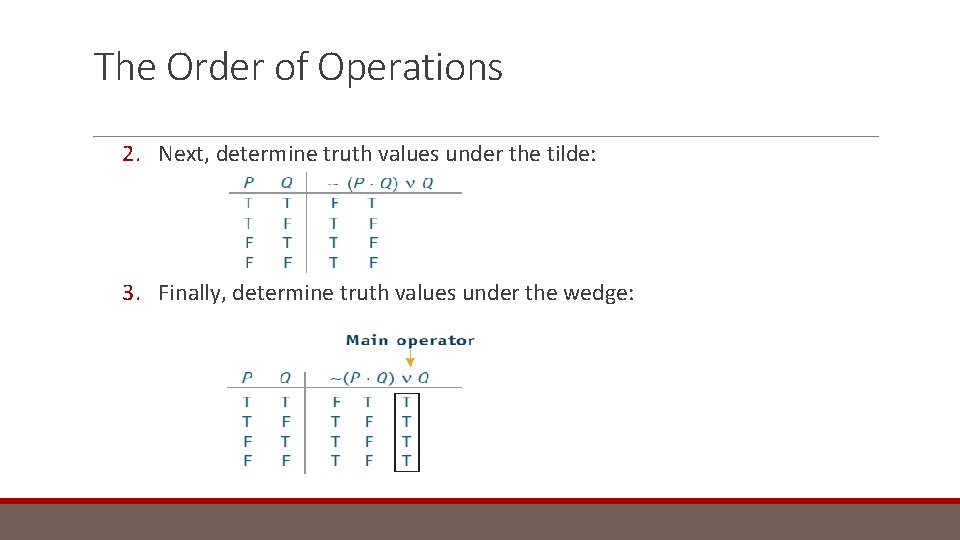The Order of Operations 2. Next, determine truth values under the tilde: 3. Finally, determine truth values under the wedge: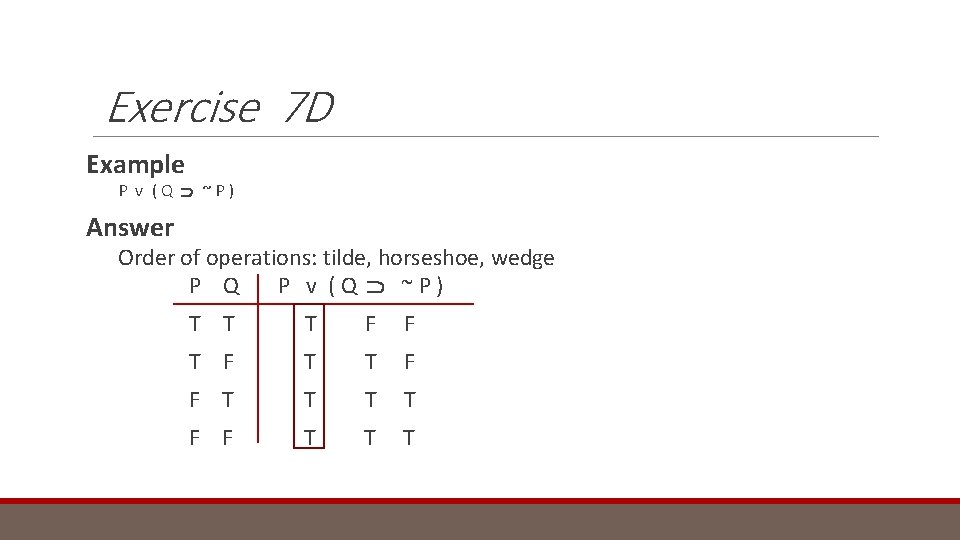Exercise 7 D Example P v (Q ~P) Answer Order of operations: tilde, horseshoe, wedge P Q P v (Q ~P) T T T F F T T TWe are skipping 7 E and 7 F!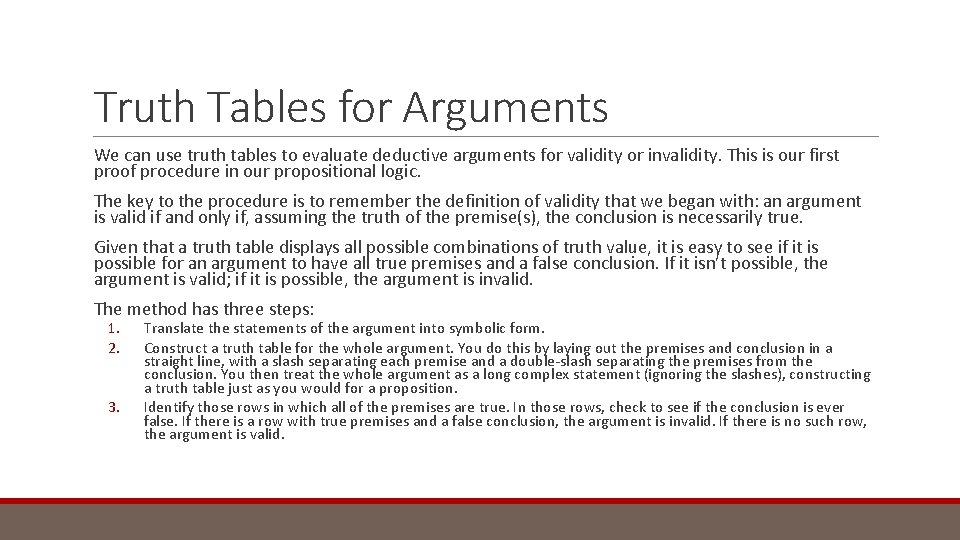Truth Tables for Arguments We can use truth tables to evaluate deductive arguments for validity or invalidity. This is our first proof procedure in our propositional logic. The key to the procedure is to remember the definition of validity that we began with: an argument is valid if and only if, assuming the truth of the premise(s), the conclusion is necessarily true. Given that a truth table displays all possible combinations of truth value, it is easy to see if it is possible for an argument to have all true premises and a false conclusion. If it isn’t possible, the argument is valid; if it is possible, the argument is invalid. The method has three steps: 1. 2. 3. Translate the statements of the argument into symbolic form. Construct a truth table for the whole argument. You do this by laying out the premises and conclusion in a straight line, with a slash separating each premise and a double-slash separating the premises from the conclusion. You then treat the whole argument as a long complex statement (ignoring the slashes), constructing a truth table just as you would for a proposition. Identify those rows in which all of the premises are true. In those rows, check to see if the conclusion is ever false. If there is a row with true premises and a false conclusion, the argument is invalid. If there is no such row, the argument is valid.The Procedure 1. Set up the truth table: Premise ConclusionThe Evaluation 2. Follow order of operations and main logical operator procedures. 3. Check for line(s) with true premises and a false conclusion. If no such lines exist, the argument is valid. 4. This argument is INVALID (checkmark on line 2).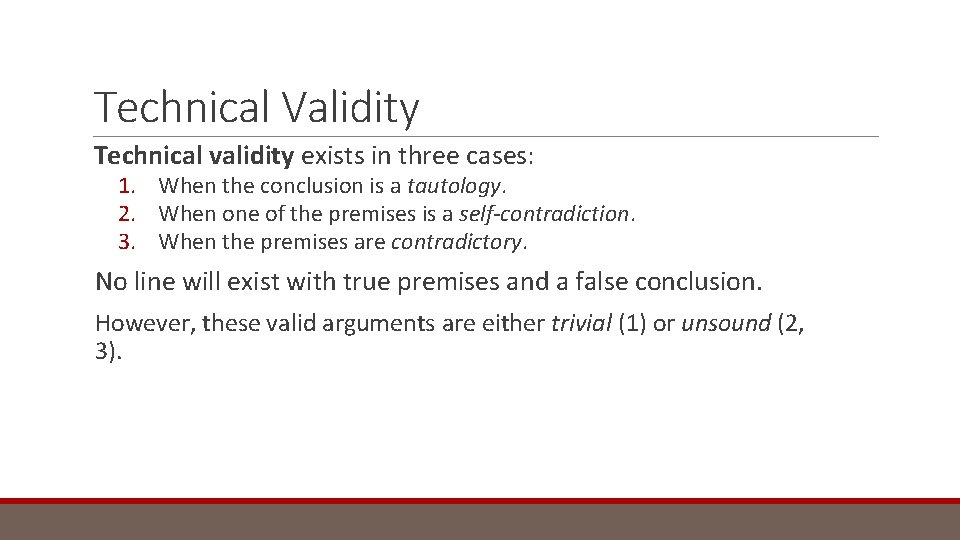Technical Validity Technical validity exists in three cases: 1. When the conclusion is a tautology. 2. When one of the premises is a self-contradiction. 3. When the premises are contradictory. No line will exist with true premises and a false conclusion. However, these valid arguments are either trivial (1) or unsound (2, 3).Exercise 7 G Example I ∼R v S ∼ S / ∼ R Answer R S ∼ R v S T T F F T T T F F T T ∼ S / ∼ R The argument is VALID: there are no lines with true premises and a false conclusion.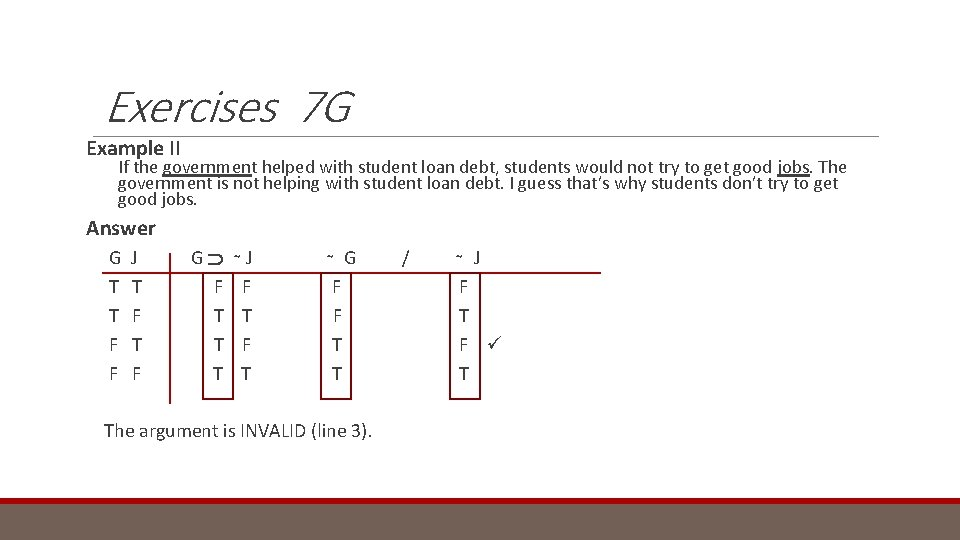Exercises 7 G Example II If the government helped with student loan debt, students would not try to get good jobs. The government is not helping with student loan debt. I guess that’s why students don’t try to get good jobs. Answer G T T F F J T F G F T T T ∼J F T ∼ G F F T T The argument is INVALID (line 3). / ∼ J F T F T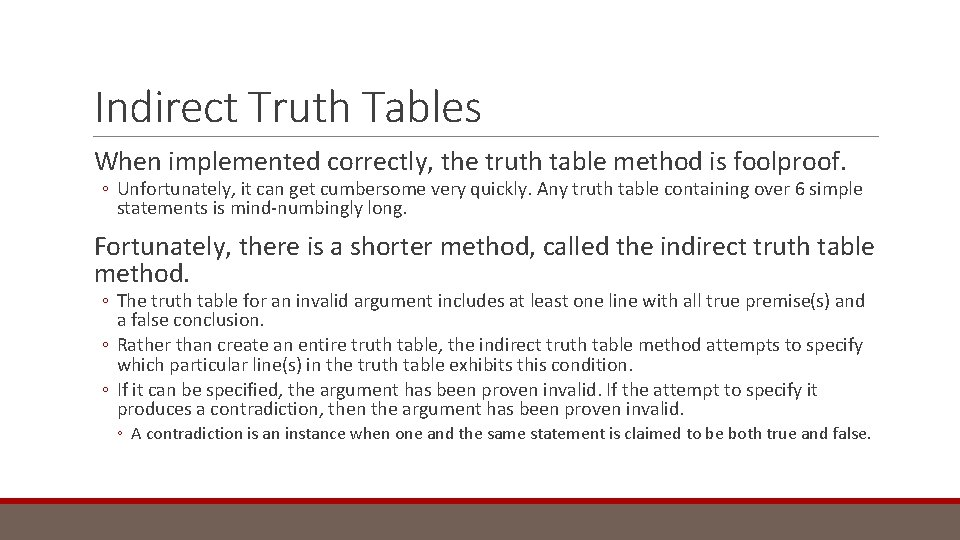Indirect Truth Tables When implemented correctly, the truth table method is foolproof. ◦ Unfortunately, it can get cumbersome very quickly. Any truth table containing over 6 simple statements is mind-numbingly long. Fortunately, there is a shorter method, called the indirect truth table method. ◦ The truth table for an invalid argument includes at least one line with all true premise(s) and a false conclusion. ◦ Rather than create an entire truth table, the indirect truth table method attempts to specify which particular line(s) in the truth table exhibits this condition. ◦ If it can be specified, the argument has been proven invalid. If the attempt to specify it produces a contradiction, then the argument has been proven invalid. ◦ A contradiction is an instance when one and the same statement is claimed to be both true and false.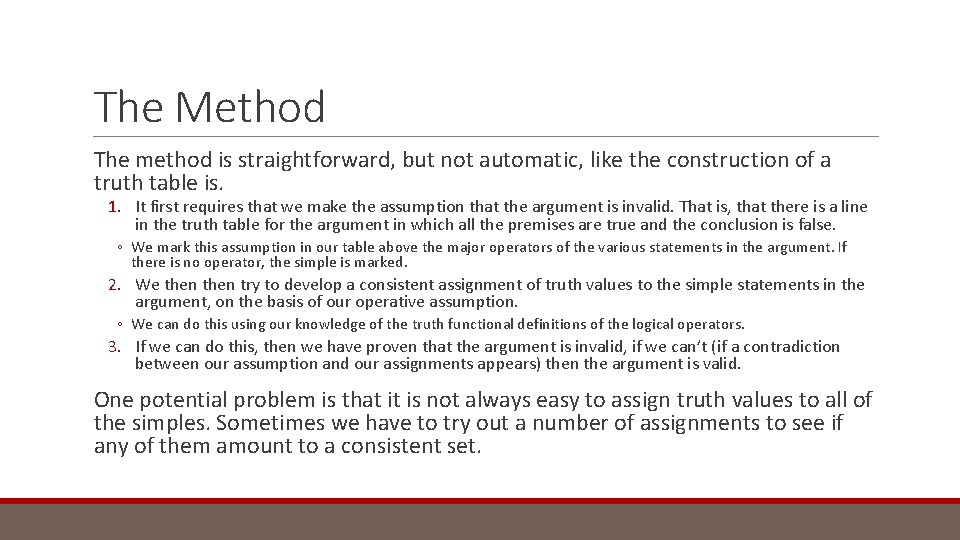The Method The method is straightforward, but not automatic, like the construction of a truth table is. 1. It first requires that we make the assumption that the argument is invalid. That is, that there is a line in the truth table for the argument in which all the premises are true and the conclusion is false. ◦ We mark this assumption in our table above the major operators of the various statements in the argument. If there is no operator, the simple is marked. 2. We then try to develop a consistent assignment of truth values to the simple statements in the argument, on the basis of our operative assumption. ◦ We can do this using our knowledge of the truth functional definitions of the logical operators. 3. If we can do this, then we have proven that the argument is invalid, if we can’t (if a contradiction between our assumption and our assignments appears) then the argument is valid. One potential problem is that it is not always easy to assign truth values to all of the simples. Sometimes we have to try out a number of assignments to see if any of them amount to a consistent set.Exercise 7 H Example (E v F) D Answer E F D F ~D (E v F) T T D F / ~(E v F) ~ D T / ~(E v F) F T F Since it is not possible for the premises to be true and the conclusion to be false, the argument is valid.Exercise 7 H Example If the Supreme Court validates the Stolen Valor Act, then veterans will lie about receiving military medals. The Supreme Court will not validate the Stolen Valor Act. Thus, veterans will not lie about receiving military medals. Answer S V S V F T T ~ S ~ V T F Since it is possible to show true premises and a false conclusion, the argument is invalid. (This example shows that S is not a necessary condition for V. It also illustrates the Fallacy of Denying the Antecedent. )